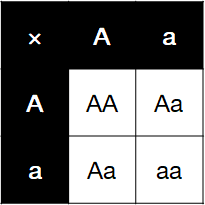# Problem: In the F2 generation of a homozygous round (AA) × homozygous wrinkled (aa) cross in peas, two round seeds are chosen at random. What is the probability that one is AA and the other Aa? The key here is the stipulation that the chosen seeds are round (i.e., AA or Aa).A) 2(2/3)(1/3)B) (1/3)2C) (2/3)2

###### FREE Expert Solution

Parent Generation: AA × aa

F1 Generation: all Aa

F2 Generation: Aa × Aa → from self-polination of F1 generation

Test Cross:###### Problem Details

In the F2 generation of a homozygous round (AA) × homozygous wrinkled (aa) cross in peas, two round seeds are chosen at random. What is the probability that one is AA and the other Aa? The key here is the stipulation that the chosen seeds are round (i.e., AA or Aa).

A) 2(2/3)(1/3)

B) (1/3)2

C) (2/3)2